# chap4_Parametric_Methods.ppt

29 Jan 2023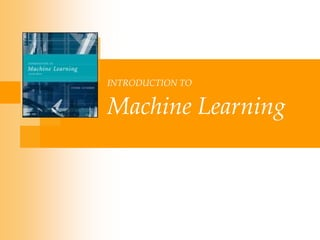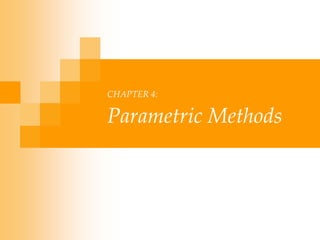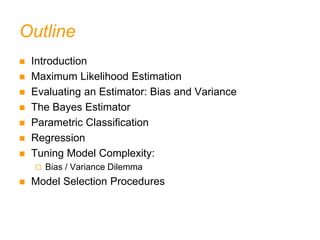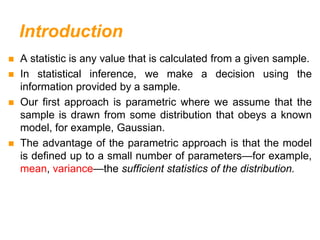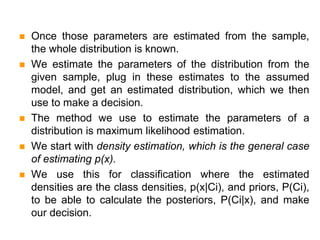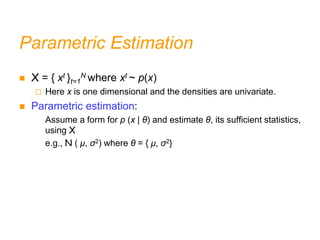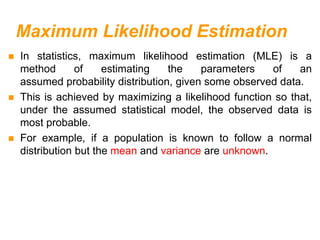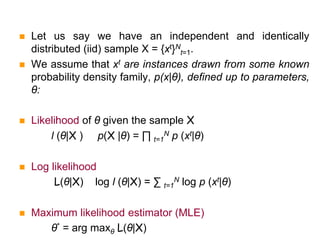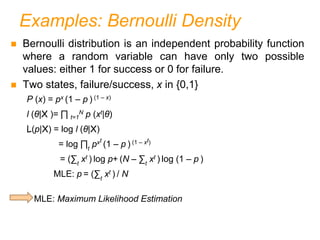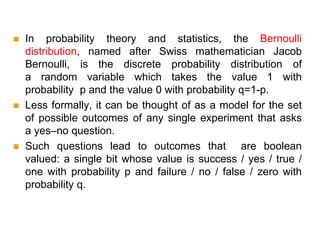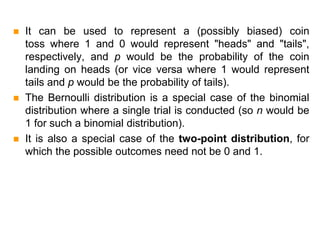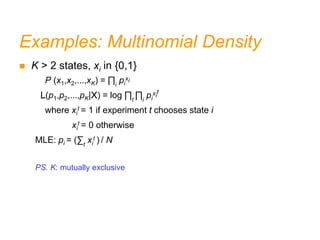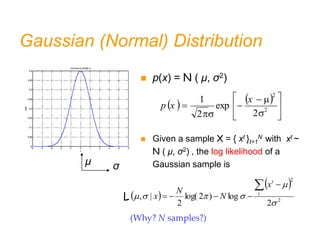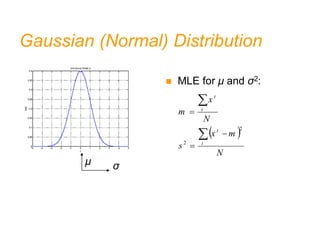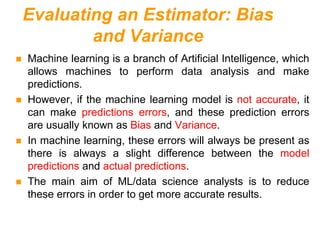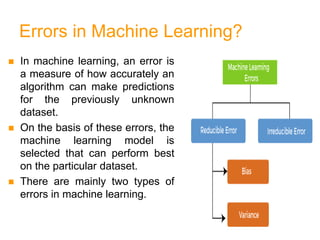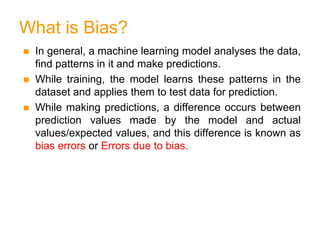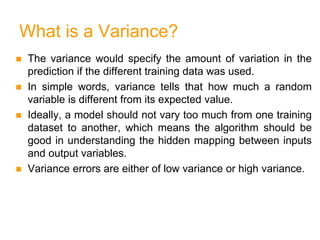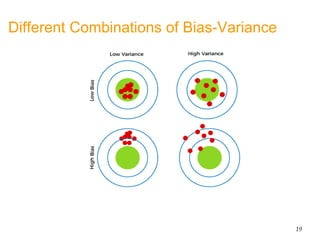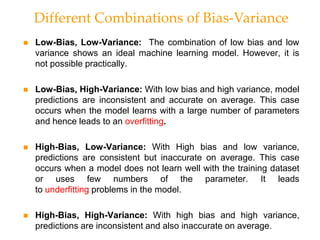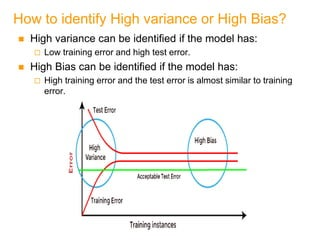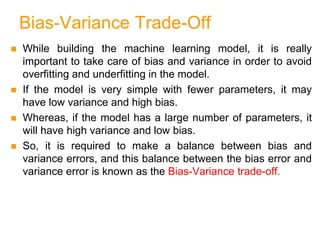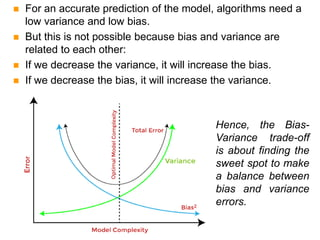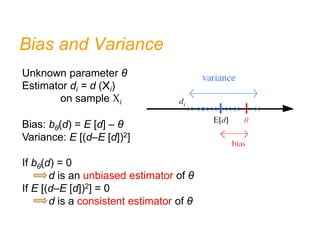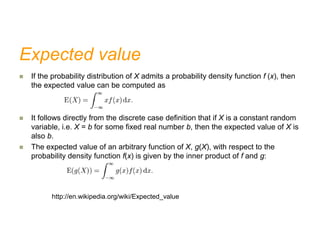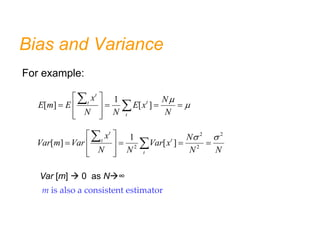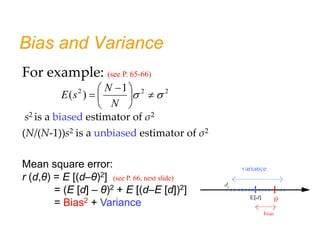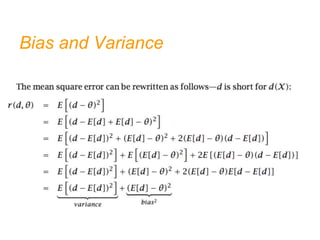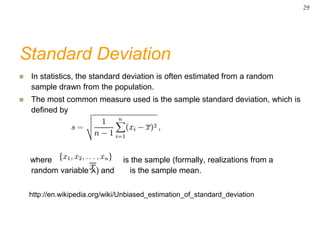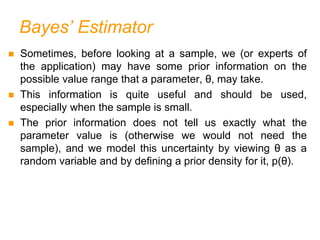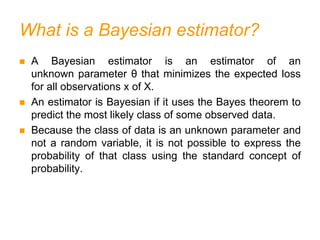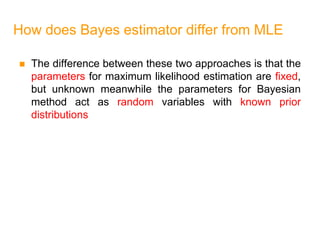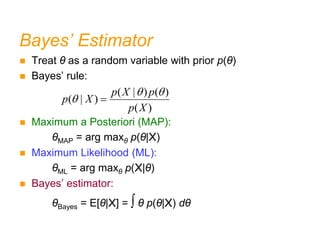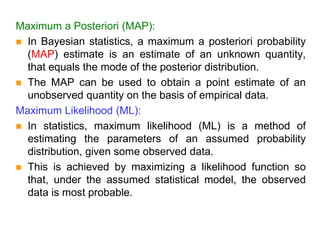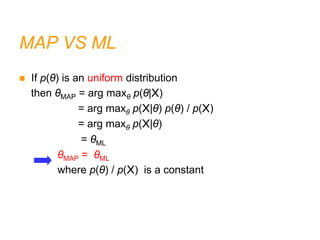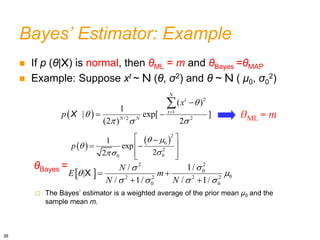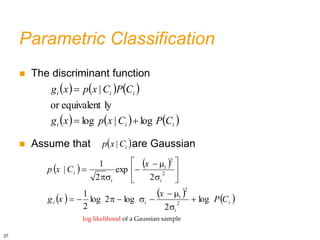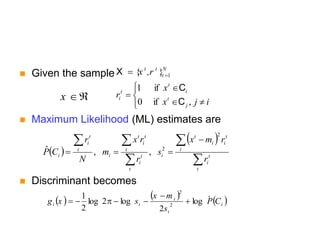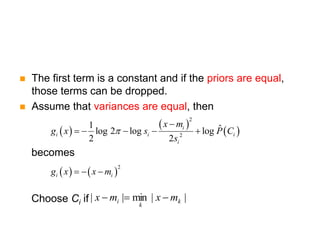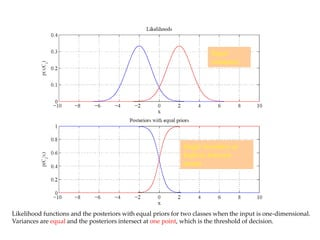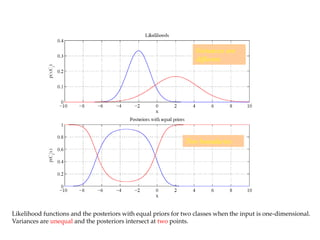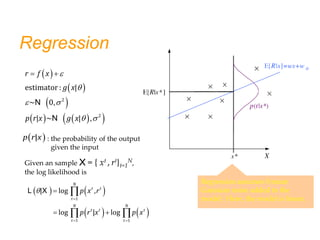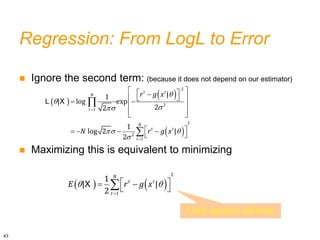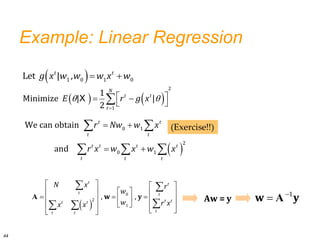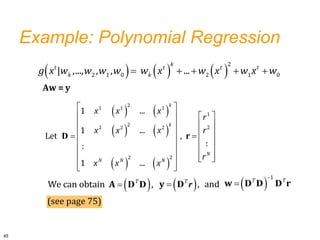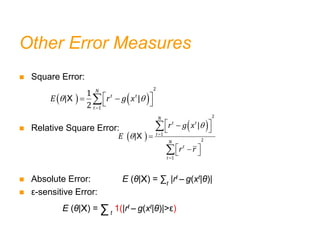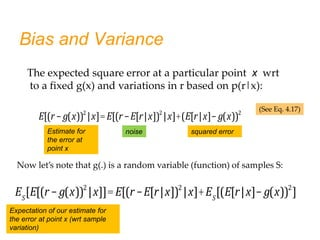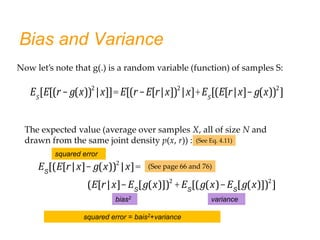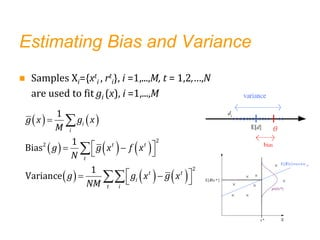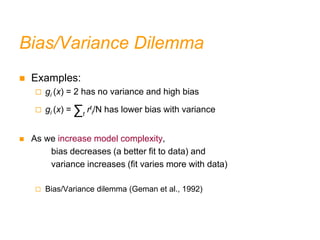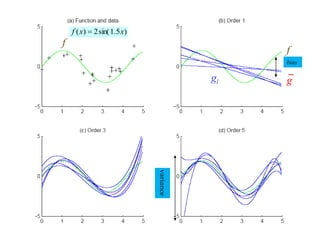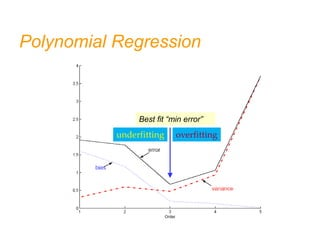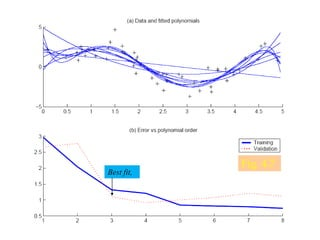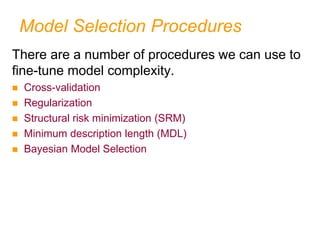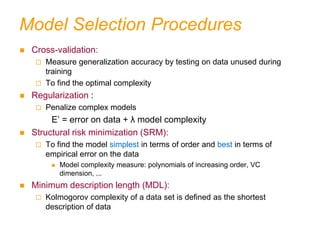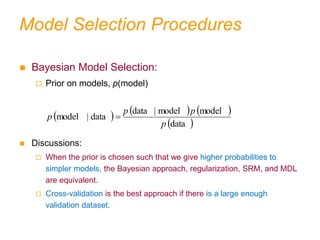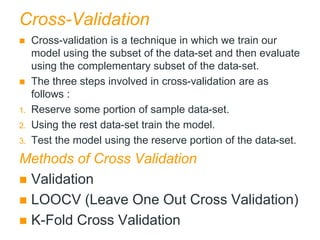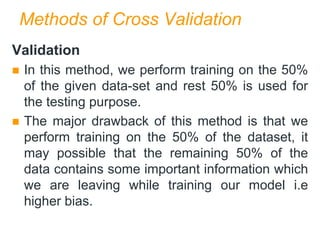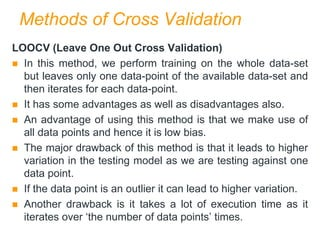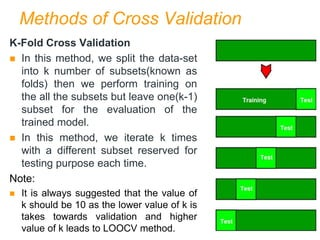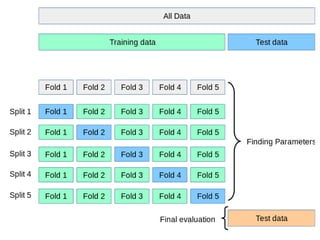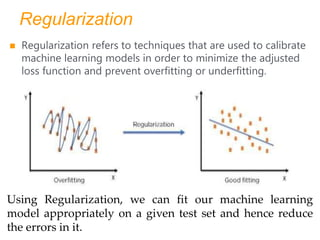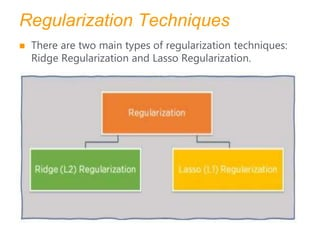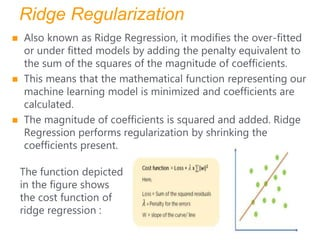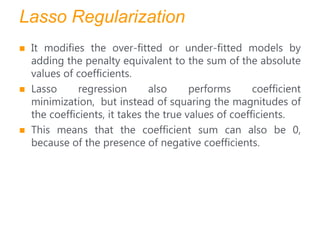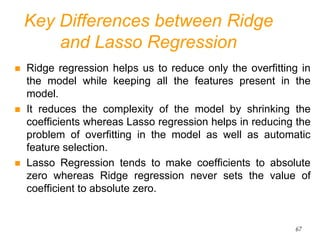1 sur 66

### chap4_Parametric_Methods.ppt

• 3. Outline  Introduction  Maximum Likelihood Estimation  Evaluating an Estimator: Bias and Variance  The Bayes Estimator  Parametric Classification  Regression  Tuning Model Complexity:  Bias / Variance Dilemma  Model Selection Procedures
• 4. Introduction  A statistic is any value that is calculated from a given sample.  In statistical inference, we make a decision using the information provided by a sample.  Our first approach is parametric where we assume that the sample is drawn from some distribution that obeys a known model, for example, Gaussian.  The advantage of the parametric approach is that the model is defined up to a small number of parameters—for example, mean, variance—the sufficient statistics of the distribution.
• 5.  Once those parameters are estimated from the sample, the whole distribution is known.  We estimate the parameters of the distribution from the given sample, plug in these estimates to the assumed model, and get an estimated distribution, which we then use to make a decision.  The method we use to estimate the parameters of a distribution is maximum likelihood estimation.  We start with density estimation, which is the general case of estimating p(x).  We use this for classification where the estimated densities are the class densities, p(x|Ci), and priors, P(Ci), to be able to calculate the posteriors, P(Ci|x), and make our decision.
• 6. Parametric Estimation  X = { xt }t=1 N where xt ~ p(x)  Here x is one dimensional and the densities are univariate.  Parametric estimation: Assume a form for p (x | θ) and estimate θ, its sufficient statistics, using X e.g., N ( μ, σ2) where θ = { μ, σ2}
• 7. Maximum Likelihood Estimation  In statistics, maximum likelihood estimation (MLE) is a method of estimating the parameters of an assumed probability distribution, given some observed data.  This is achieved by maximizing a likelihood function so that, under the assumed statistical model, the observed data is most probable.  For example, if a population is known to follow a normal distribution but the mean and variance are unknown.
• 8.  Let us say we have an independent and identically distributed (iid) sample X = {xt}N t=1.  We assume that xt are instances drawn from some known probability density family, p(x|θ), defined up to parameters, θ:  Likelihood of θ given the sample X l (θ|X ) p(X |θ) = ∏ t=1 N p (xt|θ)  Log likelihood L(θ|X) log l (θ|X) = ∑ t=1 N log p (xt|θ)  Maximum likelihood estimator (MLE) θ* = arg maxθ L(θ|X)
• 9. Examples: Bernoulli Density  Bernoulli distribution is an independent probability function where a random variable can have only two possible values: either 1 for success or 0 for failure.  Two states, failure/success, x in {0,1} P (x) = px (1 – p ) (1 – x) l (θ|X )= ∏ t=1 N p (xt|θ) L(p|X) = log l (θ|X) = log ∏t pxt (1 – p ) (1 – xt) = (∑t xt ) log p+ (N – ∑t xt ) log (1 – p ) MLE: p = (∑t xt ) / N MLE: Maximum Likelihood Estimation
• 10.  In probability theory and statistics, the Bernoulli distribution, named after Swiss mathematician Jacob Bernoulli, is the discrete probability distribution of a random variable which takes the value 1 with probability p and the value 0 with probability q=1-p.  Less formally, it can be thought of as a model for the set of possible outcomes of any single experiment that asks a yes–no question.  Such questions lead to outcomes that are boolean valued: a single bit whose value is success / yes / true / one with probability p and failure / no / false / zero with probability q.
• 11.  It can be used to represent a (possibly biased) coin toss where 1 and 0 would represent "heads" and "tails", respectively, and p would be the probability of the coin landing on heads (or vice versa where 1 would represent tails and p would be the probability of tails).  The Bernoulli distribution is a special case of the binomial distribution where a single trial is conducted (so n would be 1 for such a binomial distribution).  It is also a special case of the two-point distribution, for which the possible outcomes need not be 0 and 1.
• 12. Examples: Multinomial Density  K > 2 states, xi in {0,1} P (x1,x2,...,xK) = ∏i pi xi L(p1,p2,...,pK|X) = log ∏t ∏i pi xi t where xi t = 1 if experiment t chooses state i xi t = 0 otherwise MLE: pi = (∑t xi t ) / N PS. K: mutually exclusive
• 13. Gaussian (Normal) Distribution                 2 2 2 exp 2 1 x - x p  p(x) = N ( μ, σ2)  Given a sample X = { xt }t=1 N with xt ~ N ( μ, σ2) , the log likelihood of a Gaussian sample is μ σ                  2 2 2 exp 2 1 x x p     2 2 2 log ) 2 log( 2 | ,             t t x N N x L (Why? N samples?)
• 14. Gaussian (Normal) Distribution                 2 2 2 exp 2 1 x - x p  MLE for μ and σ2: μ σ   N m x s N x m t t t t      2 2
• 15. Evaluating an Estimator: Bias and Variance  Machine learning is a branch of Artificial Intelligence, which allows machines to perform data analysis and make predictions.  However, if the machine learning model is not accurate, it can make predictions errors, and these prediction errors are usually known as Bias and Variance.  In machine learning, these errors will always be present as there is always a slight difference between the model predictions and actual predictions.  The main aim of ML/data science analysts is to reduce these errors in order to get more accurate results.
• 16. Errors in Machine Learning?  In machine learning, an error is a measure of how accurately an algorithm can make predictions for the previously unknown dataset.  On the basis of these errors, the machine learning model is selected that can perform best on the particular dataset.  There are mainly two types of errors in machine learning.
• 17. What is Bias?  In general, a machine learning model analyses the data, find patterns in it and make predictions.  While training, the model learns these patterns in the dataset and applies them to test data for prediction.  While making predictions, a difference occurs between prediction values made by the model and actual values/expected values, and this difference is known as bias errors or Errors due to bias.
• 18. What is a Variance?  The variance would specify the amount of variation in the prediction if the different training data was used.  In simple words, variance tells that how much a random variable is different from its expected value.  Ideally, a model should not vary too much from one training dataset to another, which means the algorithm should be good in understanding the hidden mapping between inputs and output variables.  Variance errors are either of low variance or high variance.
• 19. Different Combinations of Bias-Variance 19
• 20.  Low-Bias, Low-Variance: The combination of low bias and low variance shows an ideal machine learning model. However, it is not possible practically.  Low-Bias, High-Variance: With low bias and high variance, model predictions are inconsistent and accurate on average. This case occurs when the model learns with a large number of parameters and hence leads to an overfitting.  High-Bias, Low-Variance: With High bias and low variance, predictions are consistent but inaccurate on average. This case occurs when a model does not learn well with the training dataset or uses few numbers of the parameter. It leads to underfitting problems in the model.  High-Bias, High-Variance: With high bias and high variance, predictions are inconsistent and also inaccurate on average. Different Combinations of Bias-Variance
• 21. How to identify High variance or High Bias?  High variance can be identified if the model has:  Low training error and high test error.  High Bias can be identified if the model has:  High training error and the test error is almost similar to training error.
• 22. Bias-Variance Trade-Off  While building the machine learning model, it is really important to take care of bias and variance in order to avoid overfitting and underfitting in the model.  If the model is very simple with fewer parameters, it may have low variance and high bias.  Whereas, if the model has a large number of parameters, it will have high variance and low bias.  So, it is required to make a balance between bias and variance errors, and this balance between the bias error and variance error is known as the Bias-Variance trade-off.
• 23.  For an accurate prediction of the model, algorithms need a low variance and low bias.  But this is not possible because bias and variance are related to each other:  If we decrease the variance, it will increase the bias.  If we decrease the bias, it will increase the variance. Hence, the Bias- Variance trade-off is about finding the sweet spot to make a balance between bias and variance errors.
• 24. θ Bias and Variance Unknown parameter θ Estimator di = d (Xi) on sample Xi Bias: bθ(d) = E [d] – θ Variance: E [(d–E [d])2] If bθ(d) = 0 d is an unbiased estimator of θ If E [(d–E [d])2] = 0 d is a consistent estimator of θ
• 25. Expected value  If the probability distribution of X admits a probability density function f (x), then the expected value can be computed as  It follows directly from the discrete case definition that if X is a constant random variable, i.e. X = b for some fixed real number b, then the expected value of X is also b.  The expected value of an arbitrary function of X, g(X), with respect to the probability density function f(x) is given by the inner product of f and g: http://en.wikipedia.org/wiki/Expected_value
• 26. Bias and Variance For example: Var [m]  0 as N∞ m is also a consistent estimator 1 [ ] [ ] t t t t x N E m E E x N N N                 2 2 2 2 1 [ ] [ ] t t t t x N Var m Var Var x N N N N                
• 27. Bias and Variance For example: (see P. 65-66) s2 is a biased estimator of σ2 (N/(N-1))s2 is a unbiased estimator of σ2 Mean square error: r (d,θ) = E [(d–θ)2] (see P. 66, next slide) = (E [d] – θ)2 + E [(d–E [d])2] = Bias2 + Variance 2 2 2 1 ) (            N N s E θ
• 29. Standard Deviation  In statistics, the standard deviation is often estimated from a random sample drawn from the population.  The most common measure used is the sample standard deviation, which is defined by where is the sample (formally, realizations from a random variable X) and is the sample mean. http://en.wikipedia.org/wiki/Unbiased_estimation_of_standard_deviation 29
• 30. Bayes’ Estimator  Sometimes, before looking at a sample, we (or experts of the application) may have some prior information on the possible value range that a parameter, θ, may take.  This information is quite useful and should be used, especially when the sample is small.  The prior information does not tell us exactly what the parameter value is (otherwise we would not need the sample), and we model this uncertainty by viewing θ as a random variable and by defining a prior density for it, p(θ).
• 31. What is a Bayesian estimator?  A Bayesian estimator is an estimator of an unknown parameter θ that minimizes the expected loss for all observations x of X.  An estimator is Bayesian if it uses the Bayes theorem to predict the most likely class of some observed data.  Because the class of data is an unknown parameter and not a random variable, it is not possible to express the probability of that class using the standard concept of probability.
• 32. How does Bayes estimator differ from MLE  The difference between these two approaches is that the parameters for maximum likelihood estimation are fixed, but unknown meanwhile the parameters for Bayesian method act as random variables with known prior distributions
• 33. Bayes’ Estimator  Treat θ as a random variable with prior p(θ)  Bayes’ rule:  Maximum a Posteriori (MAP): θMAP = arg maxθ p(θ|X)  Maximum Likelihood (ML): θML = arg maxθ p(X|θ)  Bayes’ estimator: θBayes = E[θ|X] = ∫ θ p(θ|X) dθ ( | ) ( ) ( | ) ( ) p X p p X p X    
• 34. Maximum a Posteriori (MAP):  In Bayesian statistics, a maximum a posteriori probability (MAP) estimate is an estimate of an unknown quantity, that equals the mode of the posterior distribution.  The MAP can be used to obtain a point estimate of an unobserved quantity on the basis of empirical data. Maximum Likelihood (ML):  In statistics, maximum likelihood (ML) is a method of estimating the parameters of an assumed probability distribution, given some observed data.  This is achieved by maximizing a likelihood function so that, under the assumed statistical model, the observed data is most probable.
• 35. MAP VS ML  If p(θ) is an uniform distribution then θMAP = arg maxθ p(θ|X) = arg maxθ p(X|θ) p(θ) / p(X) = arg maxθ p(X|θ) = θML θMAP = θML where p(θ) / p(X) is a constant
• 36. Bayes’ Estimator: Example  If p (θ|X) is normal, then θML = m and θBayes =θMAP  Example: Suppose xt ~ N (θ, σ2) and θ ~ N ( μ0, σ0 2) θBayes =  The Bayes’ estimator is a weighted average of the prior mean μ0 and the sample mean m.   2 2 0 0 2 2 2 2 0 0 1/ / | / 1/ / 1/ N E m N N             X   2 1 /2 2 ( ) 1 | exp[ ] (2 ) 2 N t t N N x p           X     2 0 2 0 0 1 exp 2 2 p                 θML = m 36
• 37. Parametric Classification  The discriminant function  Assume that are Gaussian             i i i i i i C P C x p x g C P C x p x g log | log ly equivalent or |              i i i i i i i i i C P x x g x C x p log 2 log 2 log 2 1 2 exp 2 1 | 2 2 2 2                          i C x p | 37 log likelihood of a Gaussian sample
• 38.  Given the sample  Maximum Likelihood (ML) estimates are  Discriminant becomes N t t t ,r x 1 } {   X   x          , if 0 if 1 i j x x r j t i t t i C C              t t i t t i i t i t t i t t i t i t t i i r r m x s r r x m N r C P 2 2 , , ˆ       i i i i i C P̂ s m x s x g log 2 log 2 log 2 1 2 2       
• 39.  The first term is a constant and if the priors are equal, those terms can be dropped.  Assume that variances are equal, then becomes Choose Ci if       2 2 1 ˆ log 2 log log 2 2 i i i i i x m g x s P C s            2 i i g x x m    | | min | | k k i m x m x   
• 40. Equal variances Single boundary at halfway between means Likelihood functions and the posteriors with equal priors for two classes when the input is one-dimensional. Variances are equal and the posteriors intersect at one point, which is the threshold of decision.
• 41. Variances are different Two boundaries Likelihood functions and the posteriors with equal priors for two classes when the input is one-dimensional. Variances are unequal and the posteriors intersect at two points.
• 42. Regression                     N , N , 2 2 estimator: | ~ 0 | ~ | r f x g x p r x g x                   L X 1 1 1 | log , log | log N t t t N N t t t t t p x r p r x p x Regression assumes 0 mean Gaussian noise added to the model; Here, the model is linear.   | p r x : the probability of the output given the input Given an sample X = { xt , rt}t=1 N, the log likelihood is
• 43. Regression: From LogL to Error  Ignore the second term: (because it does not depend on our estimator)  Maximizing this is equivalent to minimizing                                           L X 2 2 1 2 2 1 | 1 | log exp 2 2 1 log 2 | 2 t t N t N t t t r g x N r g x               X 2 1 1 | | 2 N t t t E r g x Least squares estimate 43
• 44. Example: Linear Regression    1 0 1 0 Let | , t t g x w w w x w            0 1 2 0 1 We can obtain and t t t t t t t t t t t r Nw w x r x w x w x                                     0 2 1 , , t t t t t t t t t t t N x r w w r x x x w y A   1 w y A (Exercise!!)               X 2 1 1 Minimize | | 2 N t t t E r g x 44 Aw = y
• 45. Example: Polynomial Regression            2 2 1 0 2 1 0 | ,..., , , ... k t t t t k k g x w w w w w x w x w x w                                               2 1 1 1 1 2 2 2 2 2 2 2 1 ... 1 ... Let , : : 1 ... k k N N N N x x x r r x x x r x x x r D    We can obtain , T A D D     1 T T w r D D D    , and T y D r 45 (see page 75) Aw = y
• 46. Other Error Measures  Square Error:  Relative Square Error:  Absolute Error: E (θ|X) = ∑t |rt – g(xt|θ)|  ε-sensitive Error: E (θ|X) = ∑t 1(|rt – g(xt|θ)|>ε)               X 2 1 1 | | 2 N t t t E r g x                      X 2 1 2 1 | | N t t t N t t r g x E r r
• 47. Bias and Variance (See Eq. 4.17) Now let’s note that g(.) is a random variable (function) of samples S: The expected square error at a particular point x wrt to a fixed g(x) and variations in r based on p(r|x): E[(r-g(x))2 |x]=E[(r-E[r|x])2 |x]+(E[r|x]- g(x))2 noise squared error ES [E[(r- g(x))2 |x]]=E[(r-E[r|x])2 |x]+ES [(E[r|x]- g(x))2 ] Estimate for the error at point x Expectation of our estimate for the error at point x (wrt sample variation)
• 48. Bias and Variance ES [(E[r|x]- g(x))2 |x]= (E[r|x]-ES [g(x)])2 +ES [(g(x)-ES [g(x)])2 ] bias2 variance The expected value (average over samples X, all of size N and drawn from the same joint density p(x, r)) : (See Eq. 4.11) (See page 66 and 76) squared error squared error = bais2+variance Now let’s note that g(.) is a random variable (function) of samples S: ES [E[(r- g(x))2 |x]]=E[(r-E[r|x])2 |x]+ES [(E[r|x]- g(x))2 ]
• 49. Estimating Bias and Variance  Samples Xi={xt i , rt i}, i =1,...,M, t = 1,2,…,N are used to fit gi (x), i =1,...,M                                 2 2 2 1 1 Bias 1 Variance i i t t t t t i t i g x g x M g g x f x N g g x g x NM θ
• 50. Bias/Variance Dilemma  Examples:  gi (x) = 2 has no variance and high bias  gi (x) = ∑t rt i/N has lower bias with variance  As we increase model complexity, bias decreases (a better fit to data) and variance increases (fit varies more with data)  Bias/Variance dilemma (Geman et al., 1992)
• 52. Polynomial Regression Best fit “min error” overfitting underfitting
• 54. Model Selection Procedures There are a number of procedures we can use to fine-tune model complexity.  Cross-validation  Regularization  Structural risk minimization (SRM)  Minimum description length (MDL)  Bayesian Model Selection
• 55. Model Selection Procedures  Cross-validation:  Measure generalization accuracy by testing on data unused during training  To find the optimal complexity  Regularization :  Penalize complex models E’ = error on data + λ model complexity  Structural risk minimization (SRM):  To find the model simplest in terms of order and best in terms of empirical error on the data  Model complexity measure: polynomials of increasing order, VC dimension, ...  Minimum description length (MDL):  Kolmogorov complexity of a data set is defined as the shortest description of data
• 56. Model Selection Procedures  Bayesian Model Selection:  Prior on models, p(model)  Discussions:  When the prior is chosen such that we give higher probabilities to simpler models, the Bayesian approach, regularization, SRM, and MDL are equivalent.  Cross-validation is the best approach if there is a large enough validation dataset.         data model model | data data | model p p p p 
• 57. Cross-Validation  Cross-validation is a technique in which we train our model using the subset of the data-set and then evaluate using the complementary subset of the data-set.  The three steps involved in cross-validation are as follows : 1. Reserve some portion of sample data-set. 2. Using the rest data-set train the model. 3. Test the model using the reserve portion of the data-set. Methods of Cross Validation  Validation  LOOCV (Leave One Out Cross Validation)  K-Fold Cross Validation
• 58. Methods of Cross Validation Validation  In this method, we perform training on the 50% of the given data-set and rest 50% is used for the testing purpose.  The major drawback of this method is that we perform training on the 50% of the dataset, it may possible that the remaining 50% of the data contains some important information which we are leaving while training our model i.e higher bias.
• 59. Methods of Cross Validation LOOCV (Leave One Out Cross Validation)  In this method, we perform training on the whole data-set but leaves only one data-point of the available data-set and then iterates for each data-point.  It has some advantages as well as disadvantages also.  An advantage of using this method is that we make use of all data points and hence it is low bias.  The major drawback of this method is that it leads to higher variation in the testing model as we are testing against one data point.  If the data point is an outlier it can lead to higher variation.  Another drawback is it takes a lot of execution time as it iterates over ‘the number of data points’ times.
• 60. Methods of Cross Validation K-Fold Cross Validation  In this method, we split the data-set into k number of subsets(known as folds) then we perform training on the all the subsets but leave one(k-1) subset for the evaluation of the trained model.  In this method, we iterate k times with a different subset reserved for testing purpose each time. Note:  It is always suggested that the value of k should be 10 as the lower value of k is takes towards validation and higher value of k leads to LOOCV method.
• 62. Regularization  Regularization refers to techniques that are used to calibrate machine learning models in order to minimize the adjusted loss function and prevent overfitting or underfitting. Using Regularization, we can fit our machine learning model appropriately on a given test set and hence reduce the errors in it.
• 63. Regularization Techniques  There are two main types of regularization techniques: Ridge Regularization and Lasso Regularization.
• 64. Ridge Regularization  Also known as Ridge Regression, it modifies the over-fitted or under fitted models by adding the penalty equivalent to the sum of the squares of the magnitude of coefficients.  This means that the mathematical function representing our machine learning model is minimized and coefficients are calculated.  The magnitude of coefficients is squared and added. Ridge Regression performs regularization by shrinking the coefficients present. The function depicted in the figure shows the cost function of ridge regression :
• 65. Lasso Regularization  It modifies the over-fitted or under-fitted models by adding the penalty equivalent to the sum of the absolute values of coefficients.  Lasso regression also performs coefficient minimization, but instead of squaring the magnitudes of the coefficients, it takes the true values of coefficients.  This means that the coefficient sum can also be 0, because of the presence of negative coefficients.
• 66. Key Differences between Ridge and Lasso Regression  Ridge regression helps us to reduce only the overfitting in the model while keeping all the features present in the model.  It reduces the complexity of the model by shrinking the coefficients whereas Lasso regression helps in reducing the problem of overfitting in the model as well as automatic feature selection.  Lasso Regression tends to make coefficients to absolute zero whereas Ridge regression never sets the value of coefficient to absolute zero. 67

### Notes de l'éditeur

1. S consists of {(xi,ri)} Its distribution is P(r1,..,rn|x1,…xn) P(x1,..,xn)